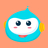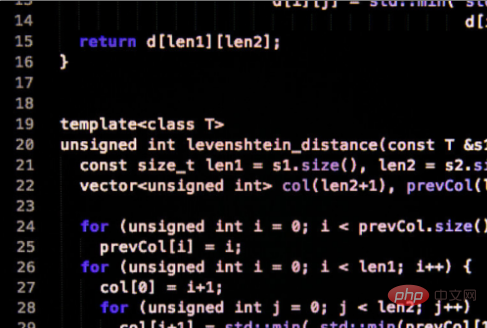• 2021-05-19 14:38:16

在c语言里二维数组在内存中的存放顺序是按行存放的，二维数组A[m][n]，这是一个m行，n列的二维数组，设a[p][q]为A的第一个元素，即二维数组的行下标从p到【m+p】，列下标从q到【n+q】即可。在c语言里二维数组元素在内存中是按行存放的。

二维数组A[m][n]，这是一个m行，n列的二维数组。设a[p][q]为A的第一个元素，即二维数组的行下标从p到m+p，列下标从q到n+q，按“行优先顺序”存储时则元素a[i][j]的地址计算为：LOC(a[i][j]) = LOC(a[p][q]) + ((i − p) * n + (j − q)) * t；

按“列优先顺序”存储时，地址计算为：LOC(a[i][j]) = LOC(a[p][q]) + ((j − q) * m + (i − p)) * t；

存放该数组至少需要的单元数为(m-p+1) * (n-q+1) * t个字节。

扩展资料：

C++动态二维数组：

以整形为例，row为行数，col为列数

int **data;//存储二维数组的指针，指向指针的指针。

date=x的地址，这样标会更好。因为sizeof(date)结果为4不可能存下二维数组。//以下实现如何申请内存

data = new int *[row];

for (int k = 0; k < row; k++)

{

data[k] = new int[col];

}//赋值跟普通二维数组一样 例如

data = 5; //将二维数组1行1列(C++中称为0行0列)赋值为5

//删除内存

for (int i = 0 ; i < row; ++i)

{

delete [] data[i]; //此处的[]不可省略

}

delete [] data;

推荐教程：《c视频教程》

更多相关内容
• 满意答案daxuezi2013.12.31采纳率：49%等级：12已帮助：6162人假设数组是a[m][n]，并且假定系统为其分配的是整块内存，那么元素在内存中存放顺序依次是：a,a.....a[n-1]，a,a.....a...

满意答案daxuezi

2013.12.31采纳率：49%    等级：12

已帮助：6162人

假设数组是a[m][n]，并且假定系统为其分配的是一整块内存，那么元素在内存中存放的顺序依次是：

a,a.....a[n-1]，

a,a.....a[n-1]，

a,a.....a[n-1]

.......

a[m-1],a[m-1].....a[m-1][n-1]

共mxn个元素。请注意，分行/分列是人为的，只是为了便于从逻辑上描述，实际上每一个元素的起始地址，从左到右，再到下一行的最左边，都是顺序增加的。

下面是用来验证上述元素顺序的一个简单小程序以及运行结果。

#include

int main()

{

int a;

int *p;

for(i=0;i<3;i++)

for(j=0;j<4;j++)

{

a[i][j]=(i+1)*(j+2);

printf("a[%d][%d]=%d\n",i,j,a[i][j]);

}

p=&a;

for (i=0;i<12;i++)

{

printf("*(p+%d)=%d\n",i,*(p+i));

}

return 0;

}

程序运行结果：

a=2

a=3

a=4

a=5

a=4

a=6

a=8

a=10

a=6

a=9

a=12

a=15

*(p+0)=2

*(p+1)=3

*(p+2)=4

*(p+3)=5

*(p+4)=4

*(p+5)=6

*(p+6)=8

*(p+7)=10

*(p+8)=6

*(p+9)=9

*(p+10)=12

*(p+11)=15

07分享举报

展开全文在c语言里二维数组在内存中的存放顺序是按行存放的，二维数组A[m][n]，这是一个m行，n列的二维数组，设a[p][q]为A的第一个元素，即二维数组的行下标从p到【m+p】，列下标从q到【n+q】即可。在c语言里二维数组元素在内存中是按行存放的。

二维数组A[m][n]，这是一个m行，n列的二维数组。设a[p][q]为A的第一个元素，即二维数组的行下标从p到m+p，列下标从q到n+q，按“行优先顺序”存储时则元素a[i][j]的地址计算为：

LOC(a[i][j]) = LOC(a[p][q]) + ((i − p) * n + (j − q)) * t；

按“列优先顺序”存储时，地址计算为：

LOC(a[i][j]) = LOC(a[p][q]) + ((j − q) * m + (i − p)) * t；

存放该数组至少需要的单元数为(m-p+1) * (n-q+1) * t个字节。

队列有几种实现方式？_后端开发

扩展资料：

C++动态二维数组：

以整形为例，row为行数，col为列数

int **data;//存储二维数组的指针，指向指针的指针。

date=x的地址，这样标会更好。因为sizeof(date)结果为4不可能存下二维数组。

//以下实现如何申请内存

data = new int *[row];

for (int k = 0; k < row; k++)

{

data[k] = new int[col];

}

//赋值跟普通二维数组一样 例如

data = 5; //将二维数组1行1列(C++中称为0行0列)赋值为5

//删除内存

for (int i = 0 ; i < row; ++i)

{

delete [] data[i]; //此处的[]不可省略

}

delete [] data;

推荐教程：《c视频教程》

展开全文• c语言里二维数组元素在内存中是按行存放的。二维数组A[m][n]，这是个m行，n列的二维数组；设a[p][q]为A的第元素，则可根据“行优先顺序”存储或“列优先顺序”存储来计算元素a[i][j]的地址。c语言里二...

在c语言里二维数组元素在内存中是按行存放的。二维数组A[m][n]，这是一个m行，n列的二维数组；设a[p][q]为A的第一个元素，则可根据“行优先顺序”存储或“列优先顺序”存储来计算元素a[i][j]的地址。在c语言里二维数组元素在内存中是按行存放的。

二维数组A[m][n]，这是一个m行，n列的二维数组。设a[p][q]为A的第一个元素，即二维数组的行下标从p到m+p，列下标从q到n+q，按“行优先顺序”存储时则元素a[i][j]的地址计算为：

LOC(a[i][j]) = LOC(a[p][q]) + ((i − p) * n + (j − q)) * t；

按“列优先顺序”存储时，地址计算为：

LOC(a[i][j]) = LOC(a[p][q]) + ((j − q) * m + (i − p)) * t；

存放该数组至少需要的单元数为(m-p+1) * (n-q+1) * t 个字节。扩展资料

C++动态二维数组：

以整形为例，row为行数，col为列数

int **data;//存储二维数组的指针(指向指针的指针。date=x的地址。这样标会更好。因为sizeof(date)结果为4不可能存下二维数组)。//以下实现如何申请内存

data = new int *[row];

for (int k = 0; k < row; k++)

{

data[k] = new int[col];

}

//赋值跟普通二维数组一样 例如

data = 5; //将二维数组1行1列(C++中称为0行0列)赋值为5

//删除内存

for (int i = 0 ; i < row; ++i)

{

delete [] data[i]; //此处的[]不可省略

}

delete [] data;

推荐教程：《C语言》

展开全文• 相关问题服装时尚流行趋势包含哪些元素中国大学MOOC: 本征半导体掺入三价元素的杂质半导体的自由电子是( )。下列说法正确的有( )。: 说法 逻辑 关系 上 数据 结构 类 线性 结构 结构 数据 逻辑 结构 数据 元素 ...
• c语言维数组元素在内存中存放顺序是什么c语言里二维数组元素在内存中是按行存放的。二维数组A[m][n]，这是个m行，n列的二维数组；设a[p][q]为A的第元素，则可根据“行优先顺序”存储或“列优先顺序...
• C语言的二维数组在内存中存放顺序发布时间：2020-06-28 18:23:49来源：亿速云阅读：708作者：Leah这期内容当中小编将会给大家带来有关C语言的二维数组在内存中存放顺序，文章内容丰富且以专业的角度为大家分析和...
• #include<...//求数组中元素个数 int i; int *p = arr;//指针变量p中存放arr[]首元素的地址 for (i = 0; i < sz; i++) //printf("%p\n",&arr[i]);打印出数组中各元素的地址，为了更直c语言
• 1 硬盘上不可能运行程序的，必须在内存中运行。...4 C语言，只能逐个地使用下标变量， 不能用个语句输出整个数组5 int a和t=a分别是定义数组长度为10和引用a数组中序号为6的元素，6不代表数组长度...
• 2012.3请编写函数fun,该函数的功能是：将M行N列的二维数组的数据,按列的顺序依次放到一维数组中。一维数 组数据的个数存放在形参n所指的存储单元。例如,若二维数组的数据为33 33 33 3344 44 44 4455 55 55 ...
• #include<stdio.h> #include<stdlib.h> void fun(int (*s),int *b,int *n,int mm,int nn); int main() { int w={{33,33,33,33},{44,44,44,44},{55,55,55,55}}, i,j;... {c语言 c++ 算法
• C++一维数组定义一维数组定义一维数组的一般格式为：类型标识符 数组名[常量表达式];例如：int a;它表示数组名为a，此数组为整型，有10个元素。关于一维数组的几点说明：1) 数组名定名规则和变量名相同，遵循...
• 《C语言一维数组教案》由会员分享，可在线阅读，更多相关《C语言一维数组教案(6页珍藏版)》请人人文库网上搜索。1、学 科：计算机科学与技术课 程：C语言程序设计课 题：一维数组课 时：2教学目标：1、掌握一维...
• Presentation on theme: "第7章 数组 本章要求： 1、掌握C语言数组在内存中的存储形式 2、掌握一维数组和二维数组的定义及使用 3、掌握使用数组的方法"— Presentation transcript:第7章 数组 本章要求： 1、掌握...
• C语言总结第七章、数组一维数组一维数组的定义一维数组的引用一维数组的初始化程序举例二维数组及多维数组二维数组的定义二维数组元素的引用二维数组元素的初始化程序举例字符数组和字符串字符数组 第七章、数组 ...C语言
• 首先我们先创建一个有10个元素一维数组，假设这个数组的元素是1~10，并将它的每个元素的地址都打印出来，观察一下其中的规律，代码书写如下： 注意这里地址的打印是要用%p，最终程序运行打印出来的结果如下...javascript es6 开发语言
• c语言高手请进1、 一维数组实验目的：掌握一维数组的定义、初始化，以及一维数组元素的下标法引用和指针法引用。实验内容：1．将数组a的10个元素后移m位，移出的m位顺序存放在数组的前位。要求：(1)数组元素...
• 将一个一维数组中相同的元素删除的只保留一个，然后按由大到小的顺序输出。
• ## [C语言] 一维数组

千次阅读 多人点赞 2021-11-25 17:54:09
这样就在内存中分配了⑤个int类型的内存空间，共 4×5=20个字节，并为它们起了个名字，叫arr。 数组中的每个元素都有个序号，这个序号从0开始，而不是从我们熟悉的1开始，称为下标（Index）。使用数组元素时，...c语言 开发语言 后端
• 存放在内存中的程序和数据都有个地址，也就是说，个函数也有自己的内存地址。2.当定义个变量时，系统就分配个带有唯一地址的存储单元来存储这个变量。比如：chara ='A'; // A的ASCII值为65i...
• ## C++实现一维数组

热门讨论 2021-12-14 17:06:02
//数组元素的地址 int *pBase; //数组存放的最大元素个数 int length; //有效元素的个数 int cnt; } Arr; //初始化数组 void init_arr(Arr *, int); //数组末尾追加元素 bool append(Arr *, int); //...算法 数据结构
• #include <stdio.h> void reverse(int* a,int n){ int i,t; for ( i = 0;i< n/2; i++){ t=*(a+i); *(a+i)=*(a+9-i); *(a+9-i)=t; } } void main(){ int *p; int a={0,1,2,3,4,5,6,7,8,9};...c语言
• 用指针方法，将一维数组int a[n] 中元素按从小到大顺序输出,n不大于100。 输入格式： n个整数，空格隔开 输出格式： 排序后的n个数，逗号隔开。 输入样例： 12 34 56 43 7 89 81 11 33 90 输出样例： 7,11,12,33,...c语言
• /**** 需求：使用一维数组存储二叉树* 步骤：* 1、查看原始数据的个数(8个)，从而制定二叉树层级(4层)，得到满二叉树节点个数(15个)* 2、二叉树节点(15个)为一维数组，全设置为0* 3、循环遍历原始数据，第一个值为...
• 请编写函数fun,该函数的功能:将m行n列的二维数组的数据,按列的顺序依次放到一维数组中。 详细代码： #include<stdio.h> void fun(int a[], int* b); int main() { int c = { 0 }, f = { 1,4...算法 c++ c语言
• 一、一维数组1、定义一个一维数组：(1)格式：存储类别 类型标识符 数组名标识符[常量表达式](2)例子：static int a(3)注意：定义数组时数组的元素必须是常量，不能是变量，例如int n=3;int a[n] 这是不对的。2、...
• 1) 计算机会给数组分配块连续的存储空间。 2) 数组名代表数组的首地址，从首地址位置，依次存入数组元素。 3) 每个元素占用相同的字节数[字节数取决于数组类型] 4) 数组的每个元素之间的地址连续。存储...